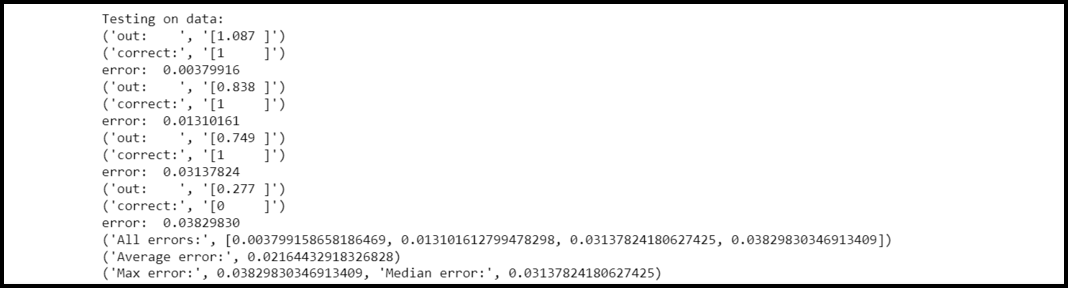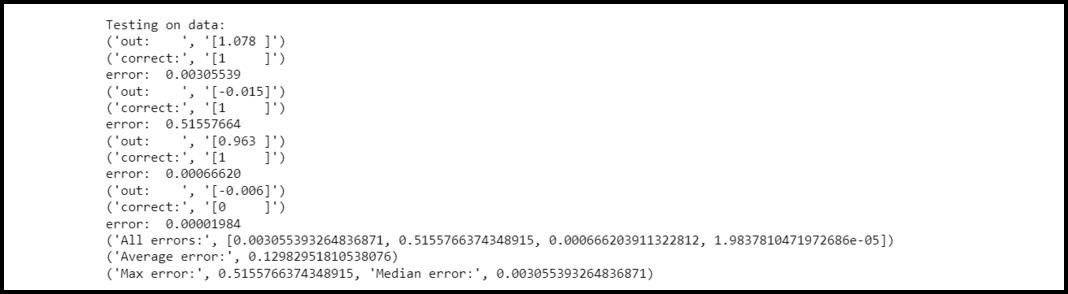GeeksforGeeks App
Open AppBrowser
Continue

# How to train a network using trainers in PyBrain

In this article, we will discuss how to train a network using trainers in PyBrain.

Network: A network consists of several modules. These modules are generally connected with connections. PyBrain provides programmers with the support of neural networks. A network can be interpreted as an acyclic directed graph where each module serves the purpose of vertex/node and connections are assumed to edge.

Dataset: A Dataset is a collection of data that is passed for testing, validating, and training on networks. A dataset is more flexible and easy to use as compared to arrays. It is quite similar to a collection of named 2d-arrays. Each task in machine learning requires specific datasets.

## Training a network using trainers in PyBrain:

PyBrain provides two trainers to test a network. These networks are discussed below,

### 1. BackpropTrainer:

This trainer is used to train parameters of the module as per the ClassificationDataSet or monitored dataset that propagates errors backward(over time).

### 2. TrainUntilConvergence:

TrainUntilConvergence is specifically used to train the module on the dataset unless it converges. While developing a neural network, the network is trained based on the data passed. To determine whether the network is significantly trained depends on the prediction of the test data tested on that network.

Example:

In this example, we have created two datasets type SupervisedDataSet. We are using the NAND data model that is given below:

The dataset used to test is given below:

## Python3

 `# Creating a dataset for testing``nand_train ``=` `SupervisedDataSet(``2``, ``1``)`` ` `# Fit input and target values to dataset``# Parameters for nand_train truth table``nand_train.addSample((``0``, ``0``), (``1``,))``nand_train.addSample((``0``, ``1``), (``1``,))``nand_train.addSample((``1``, ``1``), (``1``,))``nand_train.addSample((``1``, ``1``), (``0``,))`

The trainer used is given below:

## Python3

 `# Training the network with dataset nand_gate``trainer ``=` `BackpropTrainer(network, nand_gate)`` ` `# Iterate 100 times to train the network``for` `epoch ``in` `range``(``100``):``    ``trainer.train()``    ``trainer.testOnData(dataset``=``nand_train, verbose ``=` `True``)`

Example:

## Python3

 `# Python program to demonstrate how to train``# a network`` ` `# Importing libraries and packages``from` `pybrain.tools.shortcuts ``import` `buildNetwork``from` `pybrain.structure ``import` `TanhLayer``from` `pybrain.datasets ``import` `SupervisedDataSet``from` `pybrain.supervised.trainers ``import` `BackpropTrainer`` ` `# Establishing a network having two inputs,``# four hidden, and one output channels``network ``=` `buildNetwork(``2``, ``4``, ``1``, bias``=``True``, hiddenclass``=``TanhLayer)`` ` `# Creating a dataset that match with the ``# network input and output sizes``nand_gate ``=` `SupervisedDataSet(``2``, ``1``)`` ` `# Creating a dataset for testing``nand_train ``=` `SupervisedDataSet(``2``, ``1``)`` ` `# Fit input and target values to dataset``# Parameters for nand_train truth table``nand_gate.addSample((``0``, ``0``), (``1``,))``nand_gate.addSample((``0``, ``1``), (``1``,))``nand_gate.addSample((``1``, ``0``), (``1``,))``nand_gate.addSample((``1``, ``1``), (``0``,))`` ` `# Fit input and target values to dataset``# Parameters for nand_train truth table``nand_train.addSample((``0``, ``0``), (``1``,))``nand_train.addSample((``0``, ``1``), (``1``,))``nand_train.addSample((``1``, ``1``), (``1``,))``nand_train.addSample((``1``, ``1``), (``0``,))`` ` `# Training the network with dataset nand_gate``trainer ``=` `BackpropTrainer(network, nand_gate)`` ` `# Iterate 10 times to train the network``for` `epoch ``in` `range``(``100``):``    ``trainer.train()``    ``trainer.testOnData(dataset``=``nand_train, verbose``=``True``)`

Output:Interpretation: As you can see in the output, the test data matches with the dataset that has been used, and hence the error is 0.021 only.

Now, let us change the data and run the program again.

## Python3

 `# Creating a dataset for testing``nand_train ``=` `SupervisedDataSet(``2``, ``1``)`` ` ` ` ` ` `# Fit input and target values to dataset``# Parameters for nand_train truth table``nand_train.addSample((``0``, ``0``), (``1``,))``nand_train.addSample((``0``, ``1``), (``0``,))``nand_train.addSample((``1``, ``0``), (``1``,))``nand_train.addSample((``1``, ``1``), (``0``,))`

Example:

## Python3

 `from` `pybrain.tools.shortcuts ``import` `buildNetwork``from` `pybrain.structure ``import` `TanhLayer``from` `pybrain.datasets ``import` `SupervisedDataSet``from` `pybrain.supervised.trainers ``import` `BackpropTrainer`` ` `# Establishing a network having two inputs,``# four hidden, and one output channels``network ``=` `buildNetwork(``2``, ``4``, ``1``, bias``=``True``, hiddenclass``=``TanhLayer)`` ` `# Creating a dataset that match with the``# network input and output sizes``nand_gate ``=` `SupervisedDataSet(``2``, ``1``)`` ` `# Creating a dataset for testing``nand_train ``=` `SupervisedDataSet(``2``, ``1``)`` ` `# Fit input and target values to dataset``# Parameters for nand_train truth table``nand_gate.addSample((``0``, ``0``), (``1``,))``nand_gate.addSample((``0``, ``1``), (``0``,))``nand_gate.addSample((``1``, ``0``), (``1``,))``nand_gate.addSample((``1``, ``1``), (``0``,))`` ` `# Fit input and target values to dataset``# Parameters for nand_train truth table``nand_train.addSample((``0``, ``0``), (``1``,))``nand_train.addSample((``0``, ``1``), (``1``,))``nand_train.addSample((``1``, ``0``), (``1``,))``nand_train.addSample((``1``, ``1``), (``0``,))`` ` `# Training the network with dataset nand_gate``trainer ``=` `BackpropTrainer(network, nand_gate)`` ` `# Iterate 10 times to train the network``for` `epoch ``in` `range``(``100``):``    ``trainer.train()``    ``trainer.testOnData(dataset``=``nand_train, verbose``=``True``)`

Output:Interpretation:

As you can see in the output, the test data doesn’t exactly match with the network trainer and hence the average error is 0.129 which is greater than the previous example.

My Personal Notes arrow_drop_up